# Abstract hyperbolic differential equation

(diff) ← Older revision | Latest revision (diff) | Newer revision → (diff)

Consider the Cauchy problem for the symmetric hyperbolic system (cf. also Hyperbolic partial differential equation)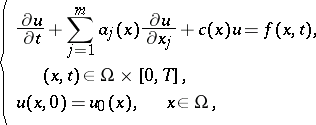with the boundary conditions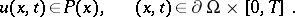Here,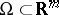is a bounded domain with smooth boundary(when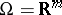, no boundary conditions are necessary), and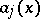,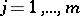, and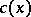are smooth functions on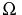with as values real matrices in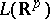, thebeing symmetric. It is assumed that the boundary matrix,, is non-singular, whereis the unit outward normal vector to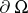. Also,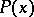denotes the maximal non-negative subspace of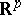with respect to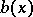, i.e.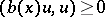,, andis not a proper subset of any other subspace ofwith this property. The function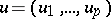is the unknown function.

One can handle this problem as the Cauchy problem for an evolution equation in a Banach space (cf. also Linear differential equation in a Banach space). Indeed, letbe the smallest closed extension in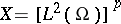of the operatordefined bywith domain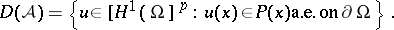Thenis the negative generator of a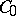semi-group on(cf. [a1], [a2]; see also Semi-group of operators). Hence, the Hille–Yoshida theorem proves the existence of a unique solutionto the Cauchy problemwhich is given in the form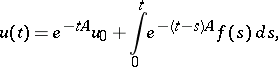Next to this idea of an abstract formulation for hyperbolic systems, the study of the linear evolution equation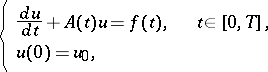was originated by T. Kato, and was developed by him and many others (cf. [a3], Chap. 7). Here,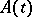denotes a given function with values in the space of closed linear operators acting in a Banach space;and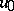are the initial data, and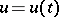is the unknown function with values in.

Among others, Kato's theorem in [a4] is fundamental: Suppose that

I)is a stable family on, in the sense that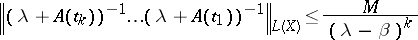for any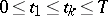and any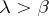with some fixedand.

II) There is a second Banach space,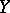, such that, andis a continuous function ofwith values in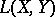.

III) There is an isomorphismfromontosuch that, with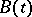a strongly continuous function ofwith values in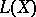. Then there is a unique solution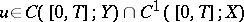, and it is given by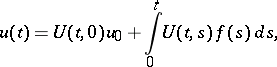,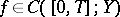, whereis a unique evolution operator. It is easily seen that III) implies, in particular, the stability ofon. Whenandare Hilbert spaces, III) can be replaced by the simpler condition [a5]:

III') There exists a positive-definite self-adjoint operatoronwith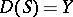such that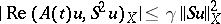for any, with some constants.

The Cauchy problem for the quasi-linear differential equation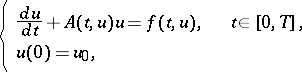has been studied by several mathematicians on the basis of results for linear problems, [a7]. Here,depends also on the unknown function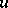. In [a6], [a7],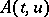, defined for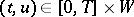, where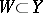is a bounded open set, is assumed to satisfy conditions similar to I)–III) and a Lipschitz condition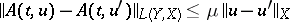with respect to. Under such conditions, the existence and uniqueness of a local solution, continuous dependence on the initial data and applications to quasi-linear hyperbolic systems have been given.

How to Cite This Entry:
Abstract hyperbolic differential equation. Encyclopedia of Mathematics. URL: http://encyclopediaofmath.org/index.php?title=Abstract_hyperbolic_differential_equation&oldid=15200
This article was adapted from an original article by A. Yagi (originator), which appeared in Encyclopedia of Mathematics - ISBN 1402006098. See original article